# Interference of waves

Also found in: Dictionary, Thesaurus, Medical, Legal.
Related to Interference of waves: Diffraction of Waves

## Interference of waves

The process whereby two or more waves of the same frequency or wavelength combine to form a wave whose amplitude is the sum of the amplitudes of the interfering waves. The interfering waves can be electromagnetic, acoustic, or water waves, or in fact any periodic disturbance.

The most striking feature of interference is the effect of adding two waves in which the trough of one wave coincides with the peak of another. If the two waves are of equal amplitude, they can cancel each other out so that the resulting amplitude is zero. This is perhaps most dramatic in sound waves; it is possible to generate acoustic waves to arrive at a person's ear so as to cancel out disturbing noise. In optics, this cancellation can occur for particular wavelengths in a situation where white light is a source. The resulting light will appear colored. This gives rise to the iridescent colors of beetles' wings and mother-of-pearl, where the substances involved are actually colorless or transparent.

To observe interference with waves generated by atomic or molecular transitions, it is necessary to use a single source and to split the light from the source into parts which can then be recombined. In this case, the amplitude and phase changes occur simultaneously in each of the parts at the same time.

The simplest technique for producing a splitting from a single source was done by T. Young in 1801 and was one of the first demonstrations of the wave nature of light. In this experiment, a narrow slit is illuminated by a source, and the light from this slit is caused to illuminate two adjacent slits. The light from these two parallel slits can interfere, and the interference can be seen by letting the light from the two slits fall on a white screen. The screen will be covered with a series of parallel fringes. The location of these fringes can be derived approximately as follows: In the illustration, S1 and S2 are the two slits separated by a distance d. Their plane is a distance l from the screen. Since the slit S0 is equidistant from S1 and S2, the intensity and phase of the light at each slit will be the same. The light falling on P from slit S1 can be represented by Eq. (1) and

(1)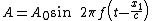(2)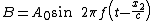from S2 by Eq. (2), where f is the frequency, t the time, c the velocity of light; x1 and x2 are the distances of P from S1 and S2, and A0 is the amplitude. This amplitude is assumed to be the same for each wave since the slits are close together, and x1 and x2 are thus nearly the same. The square of the amplitude or the intensity at P can then be written as Eq. (3).
(3)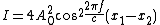In general, I is very much larger than y so that Eq. (3) can be simplified to Eq. (4).
(4)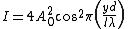Equation (4) is a maximum when Eq. (5) holds and a minimum when Eq. (6) holds, where n is an integer.
(5)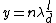(6)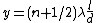Accordingly, the screen is covered with a series of light and dark bands called interference fringes. If the source behind slit S0 is white light and thus has wavelengths varying perhaps from 400 to 700 nanometers, the fringes are visible only where x1 - x2 is a few wavelengths, that is, where n is small. At large values of n, the position of the nth fringe for red light will be very different from the position for blue light, and the fringes will blend together and be washed out.

The energy carried by a wave is measured by the intensity, which is equal to the square of the amplitude. The total energy failing on the screen is not changed by the presence of interference. The energy density at a particular point is, however, drastically changed. This fact is most important for those waves of the electromagnetic spectrum which can be generated by vacuum-tube oscillators. The sources of radiation or antennas can be made to emit coherent waves which will undergo interference. This makes possible a redistribution of the radiated energy. Quite narrow beams of radiation can be produced by the proper phasing of a linear antenna array. See Interferometry

McGraw-Hill Concise Encyclopedia of Physics. © 2002 by The McGraw-Hill Companies, Inc.
Site: Follow: Share:
Open / Close